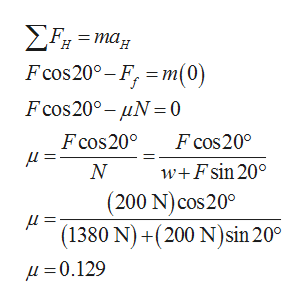# 20.0020.0°b

Question

A 1,380-N crate is being pushed across a level floor at a constant speed by a force F of 200 N at an angle of 20.0° below the horizontal, as shown in the figure a below.

(a) What is the coefficient of kinetic friction between the crate and the floor? (Enter your answer to at least three decimal places.)

(b) If the 200-N force is instead pulling the block at an angle of 20.0° above the horizontal, as shown in the figure b, what will be the acceleration of the crate? Assume that the coefficient of friction is the same as that found in part (a).    ____ m/s2

check_circleExpert Solution
Step 1

Consider the weight of the crate be w, the frictional force on the crate be Ff, the normal reaction on the crate be N, and the coefficient of the kinetic friction be µ.

Consider the free body diagrams of the crates a and b.

Step 2

(a)

Apply Newton’s second law of motion on the crate a along the vertical direction from the free body diagram of the crate a, and solve for the normal reaction on the crate.

Step 3

Apply Newton’s second law of motion on the crate a along the horizontal direction from the free body diagram of ...help_outlineImage TranscriptioncloseΣF-mα. F cos20°- F m(0) Fcos200- μN =0 Fcos20° μ F cos200 w+Fsin 20ο (200 N) cos 20 (1380 N)+(200 N)sin 20 Ν μ μ= 0.129 fullscreen

#### Want to see this answer and more?

Solutions are written by subject experts who are available 24/7. Questions are typically answered within 1 hour*

See Solution
*Response times may vary by subject and question
Tagged in

### Physics